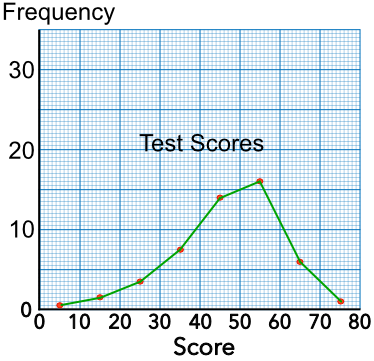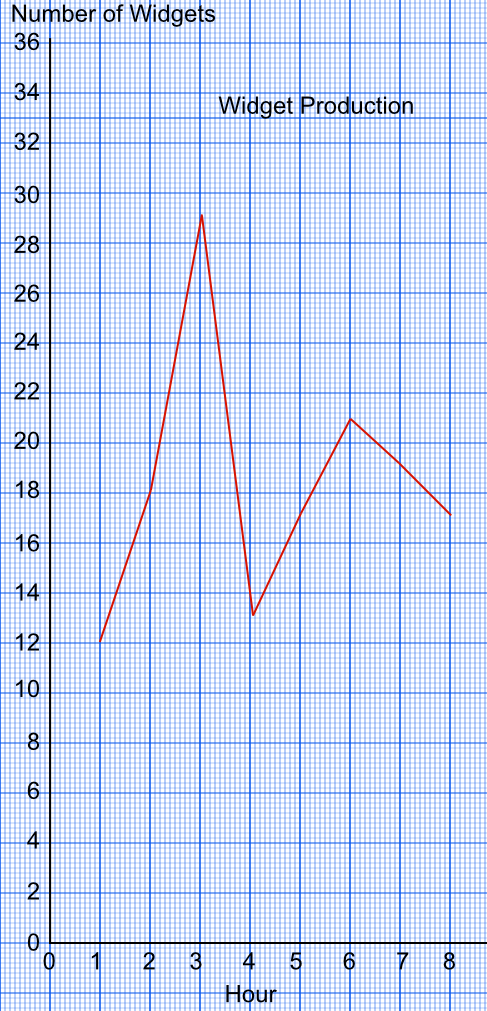Line Graphs

# Line Graphs

GCSE(F),

A Line Graph will plot frequency against a changing value, such as time. It is used for Ungrouped Data. Points should be plotted using the values provided. Only points that are plotted are joined.

This example shows the number of people in each group arriving at an exhibition:

Group SizeFrequency
1 12
2 22
3 30
4 27
5 15
6 8A Frequency Polygon is a line graph that plots the frequency of grouped data. The points plotted are the middle value of the class interval and the frequency.

This example shows the result of a test undertaken by some maths students. The frequency polygon is plotted for this data:

ScoreFrequencyMidpointPlotted Value
0 < m ≤ 10 1 5 (5, 1)
10 < m ≤ 20 3 15 (15, 3)
20 < m ≤ 30 7 25 (25, 7)
30 < m ≤ 40 15 35 (35, 15)
40 < m ≤ 50 28 45 (45, 28)
50 < m ≤ 60 32 55 (55, 32)
60 < m ≤ 70 12 65 (65, 12)
70 < m ≤ 80 2 75 (75, 2)## Examples

1. The number of widgets manufactured at a factory was plotted against the number of hours into the shift. In which hour was the highest number of widgets produced?